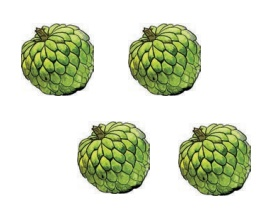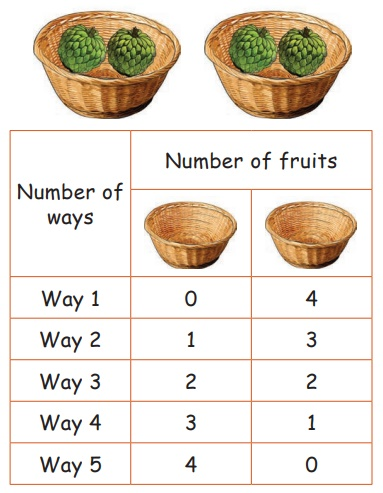Home | | Maths 2nd Std | Selection

# Selection

Teacher’s Note: Teacher may extend the activity by varying the number of objects and encourage the students to find all the possible ways.

Selection

Learn

Selection of two numbers

Let us see the possible ways of keeping 4 custard apples in two baskets.One of the ways is shown here.Key words: Selection

Teacher’s Note: Teacher may extend the activity by varying the number of objects and encourage the students to find all the possible ways.

Practice

There are two pots, one with 10 red roses and another with 5 yellow roses in the garden. List down all the possible ways of choosing ten flowers from the pots.Way 1 : 10 + 0 = 10

Way 2 : 5 + 5  = 10

Way 3 : 7 + 3 = 10

Way 4 : 6 + 4 = 10

Way 5 : 8 + 2 = 10

Way 6 : 9 + 1 = 10

Teacher’s Note: Teacher can extend the same activity and encourage the students to find the number of ways of getting the sum as 11, 12 and so on .

Tags : Information Processing | Term 1 Chapter 6 | 2nd Maths , 2nd Maths : Term 1 Unit 6 : Information Processing
Study Material, Lecturing Notes, Assignment, Reference, Wiki description explanation, brief detail
2nd Maths : Term 1 Unit 6 : Information Processing : Selection | Information Processing | Term 1 Chapter 6 | 2nd Maths